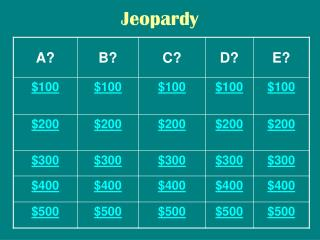# Jeopardy - PowerPoint PPT PresentationDownload PresentationJeopardy

Jeopardy
Download Presentation## Jeopardy

- - - - - - - - - - - - - - - - - - - - - - - - - - - E N D - - - - - - - - - - - - - - - - - - - - - - - - - - -
##### Presentation Transcript

1. Jeopardy

2. ANSWER This is made up of both living and nonliving things.

3. QUESTION What is: An Ecosystem

4. ANSWER True or False: Trees can live without sunlight.

5. QUESTION What is: False, trees cannot live without sunlight, water, and air. They can live though without BIRDS.

6. ANSWER • This is the part of a plant that light energy is changed into energy the plant can use.

7. QUESTION • What is: The in the LEAVES!!

8. ANSWER • This is the reason why photosynthesis cannot happen at night.

9. QUESTION • What is: Because there is no sunlight.

10. ANSWER • This is the part of the plant that takes in water from the soil.

11. QUESTION • What is: THE ROOTS!!!

12. ANSWER If I made food for myself and do not have to get it from another plant or animal, what am I?

13. QUESTION What is: A Producer.

14. ANSWER • This is why plants have leaves.

15. QUESTION • What is: to produce food. Plants get their food from their leaves.

16. ANSWER This is the primary purpose of the plant’s roots

17. QUESTION • What is: To absorb water.

18. ANSWER • This is where energy from the sun is trapped.

19. QUESTION • What is: the plant cells that have chlorophyll.

20. ANSWER These are the two things that all plants need before it can use energy from the sun to make sugar.

21. QUESTION • What are: Carbon dioxide and water.

22. ANSWER • This is how a deer gets energy.

23. QUESTION • What is: from eating plants.

24. ANSWER • TRUE OR FALSE: Grass and rabbits get the energy they need to survive from the sun.

25. QUESTION • What is: FALSE! NO WAY! FORGET THAT! Rabbits get the energy they need to survive from plants.

26. ANSWER • TRUE OR FALSE: This food chain shows the flow of energy. • SUN ---- Grass ---- Cows ---- Humans

27. QUESTION • What is: TRUE! YUP, YUP, YUP.

28. ANSWER • This is the population that would DECREASE if the number of mice greatly increased. • Sun – Green plants – Mice – Snake

29. QUESTION • What is: the green plants population would decrease.

30. ANSWER • True of False : Deforestation can be a cause for the deer population to decrease.

31. QUESTION • What is: True, deforestation can lead to the population of deer go down.

32. ANSWER • I am this _______, if I only eat plants.

33. QUESTION • What is: a herbivore.

34. ANSWER • I am this _______, if I only eat meat.

35. QUESTION • What is: a carnivore.

36. ANSWER • I am this ________, if I get both plants and animals.

37. QUESTION What is: an omnivore.

38. ANSWER • This is what happens to an oak leaf when it falls to the forest floor.

39. QUESTION What is: it is broken down by decomposers.

40. ANSWER • TRUE OR FALSE: Energy passes from plants to other organisms in a food chain.

41. QUESTION What is: ABSOLUTELY TRUE!

42. ANSWER This is the holiday that is approaching fast.

43. QUESTION • What is: Thanksgiving

44. ANSWER True or False: Decomposing leaves were once a living material.

45. QUESTION • What is: True, decomposing leaves were once living.

46. ANSWER This is what an ecosystem contains.

47. QUESTION • What is: Both living and nonliving things.

48. ANSWER • This is why plants are the basic producers for food chains.

49. QUESTION • What is: because they use the sun’s energy to make food.

50. ANSWER • TRUE OR FALSE: Only living things are part of an ecosystem.